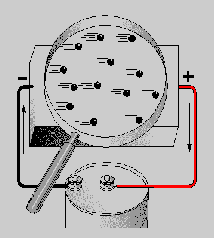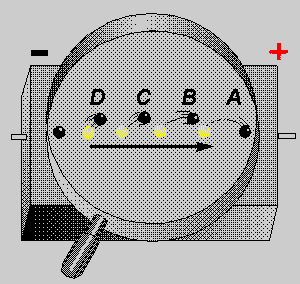Electric CurrentCustom SearchVoltage Produced by Chemical ActionMatter, Energy, and ElectricityMeasurement of CurrentELECTRIC CURRENT

It has been proven that electrons (negative charges) move through a conductor in response to an electric field. ELECTRON CURRENT FLOW will be used throughout this explanation. Electron current is defined as the directed flow of electrons. The direction of electron movement is from a region of negative potential to a region of positive potential. Therefore electric current can be said to flow from negative to positive. The direction of current flow in a material is determined by the polarity of the applied voltage. NOTE: In some electrical/electronic communities, the direction of current flow is recognized as being from positive to negative.

Q45.According to electron theory, an electric current flows from what potential to what potential?   Test Yourself

Random Drift

All materials are composed of atoms, each of which is capable of being ionized. If some form of energy, such as heat, is applied to a material, some electrons acquire sufficient energy to move to a higher energy level. As a result, some electrons are freed from their parent atom's which then becomes ions. Other forms of energy, particularly light or an electric field, will cause ionization to occur.

The number of free electrons resulting from ionization is dependent upon the quantity of energy applied to a material, as well as the atomic structure of the material. At room temperature some materials, classified as conductors, have an abundance of free electrons. Under a similar condition, materials classified as insulators have relatively few free electrons.
In a study of electric current, conductors are of major concern. Conductors are made up of atoms that contain loosely bound electrons in their outer orbits. Due to the effects of increased energy, these outermost electrons frequently break away from their atoms and freely drift throughout the material. The free electrons, also called mobile electrons, take a path that is not predictable and drift about the material in a haphazard manner. Consequently such a movement is termed RANDOM DRIFT.

It is important to emphasize that the random drift of electrons occurs in all materials. The degree of random drift is greater in a conductor than in an insulator.

Directed Drift

Associated with every charged body there is an electrostatic field. Bodies that are charged alike repel one another and bodies with unlike charges attract each other. An electron will be affected by an electrostatic field in exactly the same manner as any negatively charged body. It is repelled by a negative charge and attracted by a positive charge. If a conductor has a difference in potential impressed across it, as shown in figure 1-25, a direction is imparted to the random drift. This causes the mobile electrons to be repelled away from the negative terminal and attracted toward the positive terminal. This constitutes a general migration of electrons from one end of the conductor to the other. The directed migration of mobile electrons due to the potential difference is called DIRECTED DRIFT.Figure 1-25. - Directed drift.

The directed movement of the electrons occurs at a relatively low VELOCITY (rate of motion in a particular direction). The effect of this directed movement, however, is felt almost instantaneously, as explained by the use of figure 1-26. As a difference in potential is impressed across the conductor, the positive terminal of the battery attracts electrons from point A. Point A now has a deficiency of electrons. As a result, electrons are attracted from point B to point A. Point B has now developed an electron deficiency, therefore, it will attract electrons. This same effect occurs throughout the conductor and repeats itself from points D to C. At the same instant the positive battery terminal attracted electrons from point A, the negative terminal repelled electrons toward point D. These electrons are attracted to point D as it gives up electrons to point C. This process is continuous for as long as a difference of potential exists across the conductor. Though an individual electron moves quite slowly through the conductor, the effect of a directed drift occurs almost instantaneously. As an electron moves into the conductor at point D, an electron is leaving at point A. This action takes place at approximately the speed a light (186,000 miles Per Second).Figure 1-26. - Effect of directed drift.

Q46.The effects of directed drift take place a what rate of speed?   Test Yourself

Magnitude of Current Flow

Electric current has been defined as the directed movement of electrons. Directed drift, therefore, is current and the terms can be used interchangeably. The expression directed drift is particularly helpful in differentiating between the random and directed motion of electrons. However, CURRENT FLOW is the terminology most commonly used in indicating a directed movement of electrons.

The magnitude of current flow is directly related to the amount of energy that passes through a conductor as a result of the drift action. An increase in the number of energy carriers (the mobile electrons) or an increase in the energy of the existing mobile electrons would provide an increase in current flow. When an electric potential is impressed across a conductor, there is an increase in the velocity of the mobile electrons causing an increase in the energy of the carriers. There is also the generation of an increased number of electrons providing added carriers of energy. The additional number of free electrons is relatively small, hence the magnitude of current flow is primarily dependent on the velocity of the existing mobile electrons.

The magnitude of current flow is affected by the difference of potential in the following manner. Initially, mobile electrons are given additional energy because of the repelling and attracting electrostatic field. If the potential difference is increased, the electric field will be stronger, the amount of energy imparted to a mobile electron will be greater, and the current will be increased. If the potential difference is decreased, the strength of the field is reduced, the energy supplied to the electron is diminished, and the current is decreased.

Q47.What is the relationship of current to voltage in a circuit?   Test YourselfIntegrated Publishing, Inc. - A (SDVOSB) Service Disabled Veteran Owned Small Business